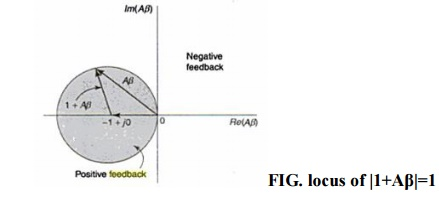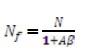Home | | Electronic Circuits II | Important Short Questions and Answers: Feed Back Amplifiers

# Important Short Questions and Answers: Feed Back Amplifiers

Electronic Circuits - Feed Back Amplifiers - Important Short Questions and Answers: Feed Back Amplifiers

FEEDBACK AMPLIFIERS

1. State the nyquist criterion to maintain the stability of negative feedback amplifier

The nyquist criterion forms the basis of a steady state method of determining whether an amplifier is stable or not.

Nyquist Criterion

The Aβ is a function of frequency. Points in the complex plane are obtained for the values of Aβ corresponding to all values of ‘f’ from -to. The locus of all these points forms a closed curve.

The criterion of  nyquist is that amplifier is unstable if  this curve encloses the point (-1+j0), and the amplifier is stable if the curve does not enclose this point.2.    Define sensitivity and desensitivity of gain in feedback amplifiers.

Sensitivity :The fractional change im amplification with feedback divided by the fractional change im amplification with out feedback is called the sensitivity of the transfer gain.Desensitivity: Desensitivity is defined as the reciprocal of sensitivity. It indicates the factor by which the voltage gain has been reduced due to feedback network.

Desensitivity factor (D) = 1+A β.

Where A = Amplifier gain.

.      β = Feedback factor.

3.           What is the effect on input and output impendence of an amplifier if it employs voltage series negative feedback?

When voltage series feedback is employed in an amplifier, its input resistance increases and output resistance decreases.

4.           Define ‘feedback factor’ of a feedback amplifier.

It is the ratio between the feedback voltages to the output voltage of the amplifier.

β = Vf/ Vo

Where β is a feedback factor (or) feedback ratio. Vf is the feedback voltage. Vo is the output voltage.

5. What is the impact of negativefeedback on noise in circuits?

When negative feedback is employed in an amlifier ,the noise is reduced.

Let N= noise without feedback

Nf = noise with feedback

The noise with feedback is given by the following relationFrom above equation it is clear that when the feedback is applied the noise is reduced by a factor (1+Aβ)

6.  What is the effect on input and output impendence of an amplifier if it employs current shunt negative feedback?

When current shunt feedback is employed in an amplifier, its input resistance decreases and output resistance increases.

7. What is return ratio of feedback amplifier?

A path of a signal from input terminals through basic amplifier, through the feedback network and back to the input terminals forms a loop. The gain of this loop is the product of –Aβ. This gain is known as loop gain or return ratio. Here the minus sign indicates the negative fedback.

8. Justify that negative feedback amplifier increases bandwidth.

When negative feedback is employed in an amlifier ,the bandwidth is increased. Let BW= bandwidth without feedback

BWf = bandwidth with feedback

The bandwidth with feedback is given by the following relation,

BWf=BW(1+Aβ)

From above equation it is clear that when the feedback is applied the bandwidth is increased is by a factor (1+Aβ)

9. Distinguish between series and shunt feedback amplifiers Series feedback:

(i). In series feedback amplifier the feedback signal is connected in series with the input signal.

(ii). It increases the input resistance.

Shunt feedback:

(i). In shunt feedback amplifier the feedback signal is connected in shunt with the input signal.

(ii). It decreases the input resistance.

10. What is current-series feedback amplifier. (or) What is transconductance amplifier?

In a current series feedback amplifier the sampled signal is a current and the feedback signal (Which is fed in series)is a voltage.

Gm =Io / Vi

Where Gm = Amplifier gain.

Io     =  Output current.

Vi    = Input voltage.

11.      List the four basic feedback topologies.

ü   Current series feedback.

ü   Current shunt feedback.

ü   Voltage series feedback

ü   Voltage shunt feedback

12.      List the characteristics of an amplifier which are modified by negative feedback.

·              It reduces the gain of an amplifier

·              It increases the stability of an amplifier.

·              It increases the bandwidth

·              It decreases noise and distortion

13. What is Feedback Amplifier? & draw the diagram.

An amplifier with feedback network is known as dfeedback amplifier. With the help of feedback network, ‘a portion of the output signal is fedback to the input & combined with the input signal to produce the desired outputs’

14.      Mention the three networks that are connected around the basic amplifier to implement the feedback concept.

The three networks that are connected around the basic amplifier to implement the feedback concept are

ü   Mixing Network

ü   Sampling Network

ü   Feedback Network

15.      What happens to the input resistance based on the type of feedback in an amplifier?

·              If the feedback signal is added to the input in series with the applied voltage, it increases the input resistance.

·              If the feedback signal is added to the input in shunt with the applied voltage, it decreases the input resistance.

16. What are the steps to be carried out for complete analysis of a feedback amplifier?

Step 1     : Identify the topology

Step 2,3: Find input and output circuit

Step 4     : Replace transistor by its h-parameter equivalent circuit

Step 5     : Find open loop voltage gain

Step 6     : Indicate V0 and Vf and calculate β

Step 7         : Calculate D, Avf, Rif, Rof and Rof.

Study Material, Lecturing Notes, Assignment, Reference, Wiki description explanation, brief detail
Electronic Circuits : Feed Back Amplifiers : Important Short Questions and Answers: Feed Back Amplifiers |